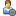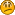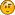###Show Posts

This section allows you to view all posts made by this member. Note that you can only see posts made in areas you currently have access to.

### Messages - Zekaria

Pages: 
1
##### Flat Earth Debate / Re: Obligate aenerobes
« on: March 19, 2015, 03:17:10 PM »
it's the force applied by aether.

oxygen kills in the same way. it might be hyperconduction that kills them

So one minute it's the force coming from the aether that's killing obligate anaerobes, and the other it is heat?  I'd hate to sound rude dude, but are you pulling explinations out of thin air?

2
##### Flat Earth Debate / Re: How do flat earthers explain satellite radio?
« on: March 19, 2015, 09:30:21 AM »

I'm from Louisville (louey-ville).  I went to Trinity High School.  Go Rocks!

Anyways, Your satellite radio works because of towers and stratellites.  It's as simple as that.

Sweet.  Where are the locations of the towers and stratellites used for satellite radio?

Why call it satellite radio to begin with if that's not what they use?

3
##### Flat Earth Debate / Re: On the subject of a curve
« on: March 19, 2015, 08:48:17 AM »
Thanks, I feel right at home4
##### Flat Earth Debate / Re: On the subject of a curve
« on: March 19, 2015, 08:27:45 AM »
Aaaaaaand I just wasted a bunch of time explaining something that should be pretty obvious.5
##### Flat Earth Debate / Re: On the subject of a curve
« on: March 19, 2015, 08:26:21 AM »
The equation I gave you is in fact a linear equation.  It has a constant slope.  I would say that most mathematicians would agree that a linear equation would give you a straight line.

Lets say we have 3 points: (0,1), (74,75), & (99,100)

If slope can be represented as M in an equation, and we assume the line is straight, we will see that slope for a linear equation is:

M = Y2  Y1

X2  X1

If we plug in the first two points [(0,1) & (74,75)] into the equation above, we will get

M1 = (75 - 1) = 1 = 1

(74 - 0)    1

Now, if the slope between points 1 & 3 or 2 & 3 are any different from what we calculated, than it will be proof that the line isnt linear.

Now, lets finish this thing and figure out if the line is in fact straight.

M2 = (100 - 1) = 1 = 1

(99 - 0)      1

M3 = (100 - 75) = 25 = 1

(99 - 74)      25

Now, do the slopes equal each other?

They do!  Who would have thought

6
##### Flat Earth Debate / Re: How do flat earthers explain satellite radio?
« on: March 19, 2015, 06:26:12 AM »
Something something aether, something something bendy waves.

7
##### Flat Earth Debate / Re: On the subject of a curve
« on: March 19, 2015, 06:19:00 AM »

Now, now, FalseProphet, don't be difficult.

y=x+1

Plug any numbers into x and you will see that you will get a straight line.

That's not a proof, it's just a formula. It's math, man, you do not get it cheap.

So tell me then, what would be proof that a mathematical straight line is actually straight?  A graph?

8
##### Flat Earth Debate / Re: On the subject of a curve
« on: March 19, 2015, 06:08:32 AM »
Well Mikey, you probably have a better understanding of math than I do haha

9
##### Flat Earth Debate / Re: On the subject of a curve
« on: March 19, 2015, 05:40:24 AM »
If curved lines can look straight, why can straight lines never look curved?Because a straight line by definition, cant be curved.
Get it right, Noriega!

Not even locally?

A mathematical straight line can never be anything but.

Oh... But can you prove that?

Now, now, FalseProphet, don't be difficult.

y=x+1

Plug any numbers into x and you will see that you will get a straight line.

Pages: